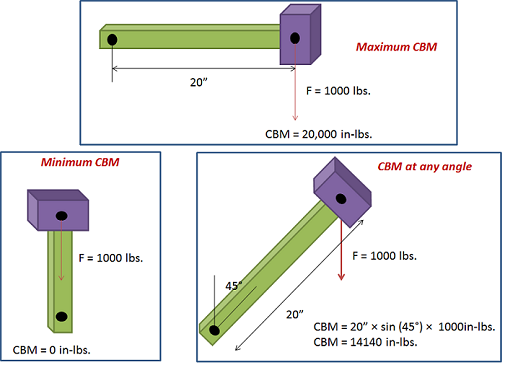# Torque and Maximum Counterbalance Moment

Torque is defined as twisting force. To calculate the torque around the rotation of a crank caused by a weight at the end of the crank, you need to multiply the weight times the horizontal distance from the center of gravity of the weight to point of rotation.

Unit of Torque:

The International System of Units, SI, (the French Système International (d’unités)), suggests using the unit newton meter (N⋅m). The unit newton meter is properly denoted N⋅m or N m. This avoids ambiguity with mN (millinewtons).

In Imperial units, “pound-force-feet” (lbf-ft), “foot-pounds-force”, “inch-pounds-force” are used. Other non-SI units of torque include “meter-kilograms-force” are also used. For all these units, the word “force” is often left out. For example, abbreviating “pound-force-foot” to simply “pound-foot” (in this case, it would be implicit that the “pound” is pound-force and not pound-mass).

### Maximum and Minimum Counterbalance Moment:

The crank generates maximum torque when it is in a horizontal position. This maximum torque is known as the Maximum Counterbalance Moment” ( maximum CBM) expressed in inch-pounds.NB: In rod pumping, the CBM is expressed in thousands of inch pounds.

When the crank is vertical, the horizontal distance between the force and the point of rotation is zero. Therefore, the torque is zero (Minimum Torque).

### Maximum Counterbalance Moment at any angle:

For any position of the crank between the minimum and the maximum torque positions, the horizontal distance between the force and the point of rotation must be calculated. To calculate it, you need to multiply the crank length times the sine of the crank angle from the vertical.

Example: if the crank angle from vertical is 45 degrees and crank length is 20 inches. The horizontal distance is: 20” × sin (45°) = 20 × 0,707 = 14,14 inches.

Assume that the weight at the center of gravity is 1000 lbs.Therefore, the torque at this position is 1000 lbs. × 7,07 in = 14140 in-lbs.